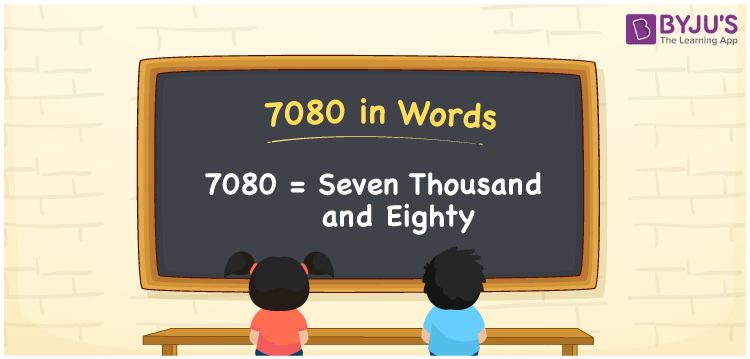# 7080 in Words

The number 7080 has a number name of seven thousand eighty. It is simple to convert the number 7080 into words using the place value of a given number. As a result, the place value chart is essential for writing numerical names. For example, if you spent Rs. 7080 on purchasing a cycle, you can write “I spent Rs. Seven thousand eighty on purchasing a cycle”. In this article, we’ll learn how to write the number 7080 in words as well as how to spell it in English.

 7080 in Words: Seven Thousand Eighty Seven Thousand Eighty in Numerical Form: 7080

## 7080 in English Words## How to Write 7080 in Words?

The following is a place value chart for the number 7080. To write the number name, determine the place value of the number first, then convert the number to words.

 Thousands Hundreds Tens Ones 7 0 8 0

Below is the expanded form of the number 7080.

= 7 × Thousand + 0 × Hundred + 8 × Ten + 0 × One

= 7 × 1000 + 8 × 10.

= 7000 + 80

= 7080

= seven thousand eighty

Hence, 7080 in words is seven thousand eighty.

The natural number 7080 appears after 7079 but just before 7081.

7080 in words – Seven thousand eighty

Is 7080 an odd number? – No

Is 7080 an even number? – Yes

Is 7080 a perfect square number? – No

Is 7080 a perfect cube number? – No

Is 7080 a prime number? – No

Is 7080 a composite number? – Yes

## Frequently Asked Questions on 7080 in Words

Q1

### How to write 7080 in words?

7080 in words is seven thousand eighty.

Q2

### Simplify 7100 – 20, and express in words.

Simplifying 7100 – 20, we get 7080. Hence, 7080 in words is seven thousand eighty.

Q3

### Convert seven thousand eighty into numbers.

Seven thousand eighty in numbers is 7080.